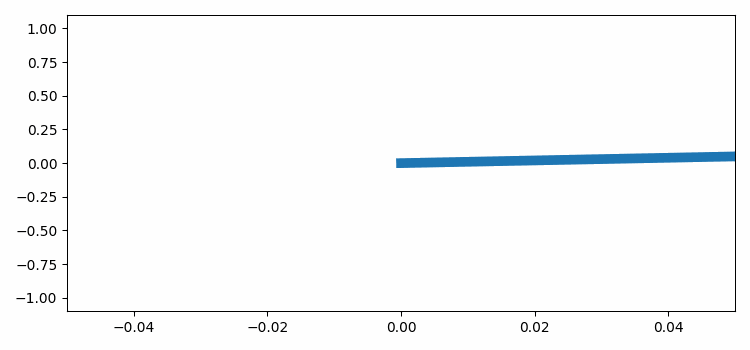# Updating the X-axis values using Matplotlib animation

To update the X-axis values using Matplotlib animation, we can take the following steps −

• Set the figure size and adjust the padding between and around the subplots.
• Create a figure and a set of subplots.
• Create x and y data points using numpy.
• Plot x and y data points using plot method on axis (ax).
• Make an animation by repeatedly calling a function animate that sets the X-axis value as per the frame.
• To display the figure, use show() method.

## Example

import matplotlib.pylab as plt
import matplotlib.animation as animation
import numpy as np

plt.rcParams["figure.figsize"] = [7.50, 3.50]
plt.rcParams["figure.autolayout"] = True

fig, ax = plt.subplots()
x = np.linspace(0, 15, 100)
y = np.sin(x)

ax.plot(x, y, lw=7)

def animate(frame):
ax.set_xlim(left=0, right=frame)

ani = animation.FuncAnimation(fig, animate, frames=10)

plt.show()

## Output